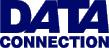The British Informatics Olympiad is the computing competition for schools and colleges. We are proud to present BIO'98.The 1998 British Informatics Olympiad exam

Question 3Question 3 Alphametics (Cryptarithms) An alphametic is an arithmetic expression where the numbers have been replaced by letters. Each number is replaced by the same letter throughout the expression, and no letter is used to represent more than one number. Furthermore, the leftmost digit of any number is not allowed to be a zero. Given an alphametic, the problem is to reconstruct the original.
 The classic puzzle is SEND + MORE = MONEY. The only solution to this problem is 9567 + 1085 = 10652. The restriction on the leftmost digit is important. It means, for example, that 8542 + 0915 = 09457 is not a valid solution. 3 (a) [24 marks] Write a program which solves addition alphametics. Your program should first accept a number n, between 3 and 6 inclusive, which will indicate the number of lines to follow. The following n lines will each contain a word of no more than 10 characters. The alphametic to be solved is the sum of the first n-1 words, to total the final word. Sample run 3 SEND MORE MONEY 9567 + 1085 = 10652 Unique Your output should consist of an example solution if you believe there to be one, or the word 'Impossible' if you do not. If you find a solution and believe it to be the only one, you should additionally print out 'Unique'.
 3 (b) [4 marks] Find two solutions to BIO + ROUND = FIRST. 3 (c) [3 marks] If you are only allowed to use a single letter, is it possible to construct an addition alphametic with a solution? If you believe it is possible give an example alphametic, and if you believe it is impossible give a brief explanation why. 3 (d) [5 marks] An alphametic is being created, where ABC and DEA are to be summed, and the total is to only use the letters A, B, C, D or E. How many possibilities (i.e. valid letter combinations) are there for the total, so that the resulting alphametic has a solution? How many different sums can be represented by such an alphametic?